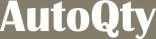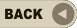Project Summary (RCC) Total Concrete Quantity (Part 1)Total Concrete Quantity (Part 2)Total Concrete Quantity (Part 3)Total Concrete Quantity (Part 4)Total Beam ReinforcementTotal Column ReinforcementTotal Slab ReinforcementTotal Foundation Reinforcement